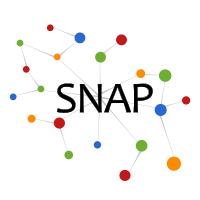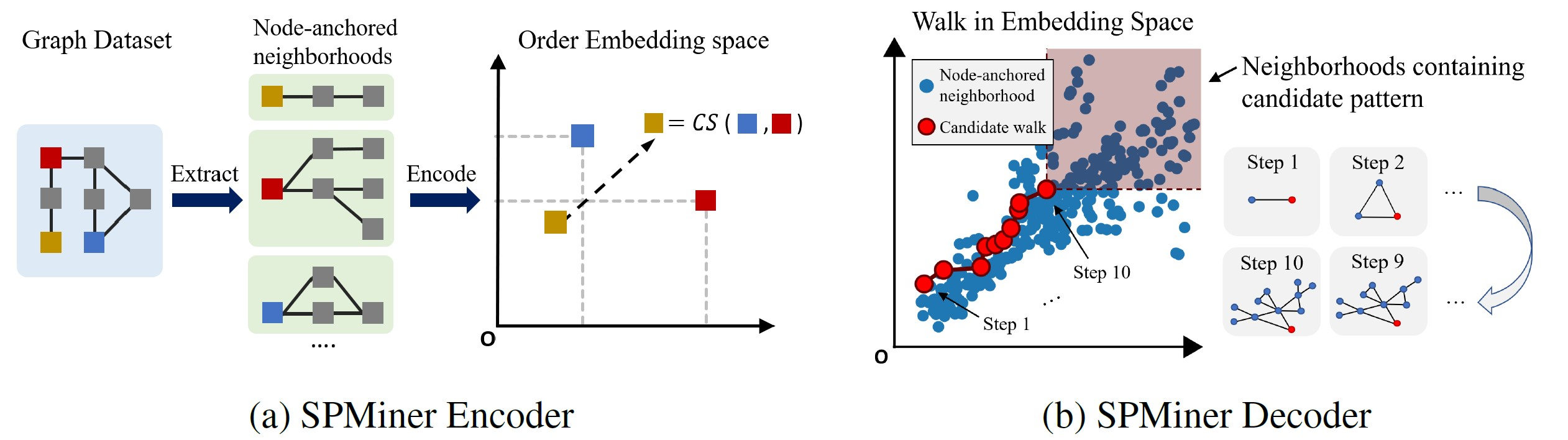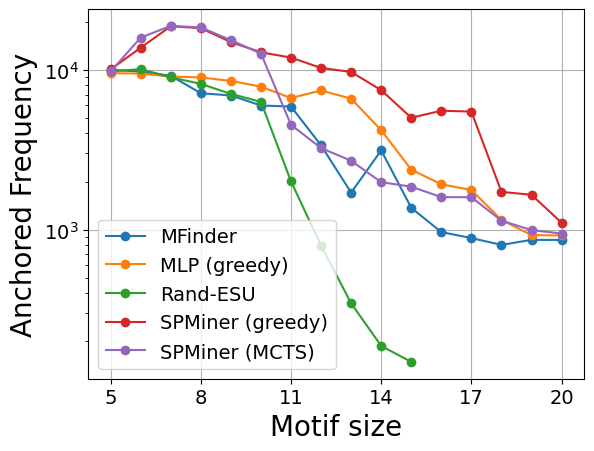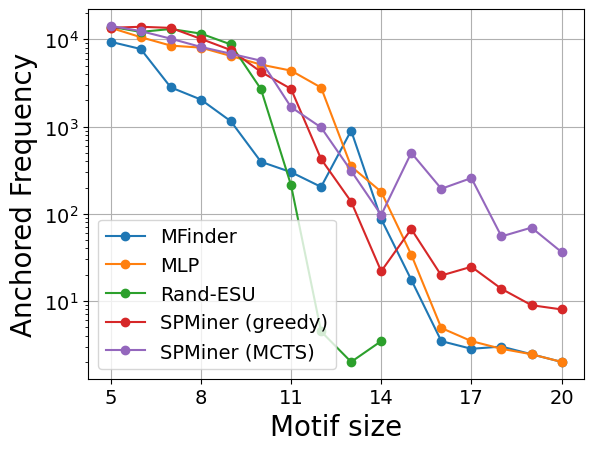# SPMiner: Frequent Subgraph Mining by Walking in Order Embedding Space

SPMiner (Subgraph Pattern Miner) is a general tool for finding frequent subgraphs in a large target graph. SPMiner integrates graph neural networks (GNNs), order embedding space, and an efficient search strategy to identify network subgraph patterns that appear most frequently in a target graph dataset.

## Motivation

Finding subgraph patterns or network motifs that frequently recur in a graph dataset is important for identifying interpretable structural properties of complex networks. Example applications include:

However, frequent subgraph mining has extremely high computational complexity. A traditional approach to motif mining is to enumerate all possible motifs Q of size up to k, usually up to 5, and then count appearances of Q in a given dataset. Frequent subgraph counting is very computationally challenging because it requires solving two intractable search problems:

• (1) Counting the frequency of a given motif Q in G.
• (2) Searching over all possible motifs Q to identify the frequent ones.
Problem (1) is NP-hard, while Problem (2) is also hard because the number of possible graphs Q increases super-exponentially with their size.

SPMiner is the first neural approach to approximately identify the most frequent subgraphs, outperforming existing heuristics and search-based approximation algorithms.

## MethodSP-Miner is a general framework using graph representation learning for identifying frequent motifs in a large target graph. It consists of two steps: an encoder for embedding subgraphs and a motif search procedure.

Encoder is an expressive graph neural network (GNN) with trainable dense skip layers. We decompose the input graph into overlapping node-anchored neighborhoods around each node. The encoder then maps these neighborhoods to points in an order embedding space. The order embedding space is trained to enforce the property that if one graph is a subgraph of another, then they are embedded to the "lower left" of each other (See part (a) for the figure above). Hence the order embedding space captures the partial ordering of graphs under the subgraph relation.

Motif Search Procedure SPMiner then reasons in the embedding space to identify frequent motifs of desired size k. SPMiner searches for a k-step walk in the embedding space that stays to the lower left of as many neighborhoods (blue dots) as possible (See part (b) of the figure above). The walk is performed by iteratively adding nodes and edges to the current motif candidate, and tracking its embedding. The number of subgraphs to the top right in the embedding space gives the frequency of the motif. Three different search procedures are explored:

• Greedy search
• Beam search
• Monte Carlo Tree Search

See paper for the mathematical definition of the frequent subgraph mining objective, and why the above algorithm can optimize for the objective.

## ExperimentsOn several real-world datasets, SPMiner outperforms both popular non-neural baselines and a multi-layer perceptron (MLP) baseline in finding frequent motifs given a desired motif size. SPMiner is especially able to find frequent large motifs (size 15+), thus better handles combinatorial explosion of search space. Datasets shown are Cox2 (left) and Enzymes (right). Baselines used are MFinder and Rand-ESU. Please refer to our paper for detailed explanations and more results.

## Code

A reference implementation of SPMiner is available on Github (https://github.com/snap-stanford/neural-subgraph-learning-GNN). The Repository Subgraph Learning is a general library to perform subgraph matching, and subgraph mining tasks, and can be extended to perform multiple tasks related to subgraph predictions (e.g. counting subgraphs).